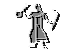# Teaching Portfolio

###Assane Lo, Ph. D
Assistant Professor
Department of Mathematics and Statistics
King Fahd University of Petroleum and Minerals

Mathematics has deep and stunning applications, but the student cannot begin to appreciate them until he has mastered a large number of preliminary steps. My goal as an instructor to is provide those steps, to encourage the student to stick to them, and to become engage in the learning process.

### Teaching Experience

The following is a description of my teaching experience as a graduate student at the University of Arizona. Over the past five years, I've had the opportunity to teach several lower division undergraduate courses as well as running review and recitation sessions for both graduate and advanced undergraduate students.

Primary Instructor

As the primary instructor for several of the math department's undergraduate courses at the University of Arizona, my duties include: preparing and presenting all course lectures, assigning and grading student projects and homework, writing and grading all course exams, and assigning final grades. The enrollment in each of these math courses is limited at 35 students. Student feedbacks are provided below.

# Courses Taught:

## University of Arizona

• Math 223: Vector Calculus (Fall 2006 and Spring 2007- 4 units)

Description: Vectors, differential and integral calculus of several variables

• Math 129: Calculus II (Spring 2006 and Fall 2005 - 3 units)

Description: Continuation of MATH 124 or MATH 125. Techniques of symbolic and numerical integration, applications of the definite integral to geometry, physics, economics, and probability; differential equations from a numerical, graphical, and algebraic point of view; modeling using differential equations, approximations by Taylor series. A graphing calculator is required for this course.

• Math 124: Calculus I (Spring 2005 - 4 units)

Description: Introduction to calculus with an emphasis on understanding and problem solving. Concepts are presented graphically and numerically as well as algebraically. Elementary functions, their properties and uses in modeling; the key concepts of derivative and definite integral; techniques of differentiation, using the derivative to understand the behavior of functions; applications to optimization problems in physics, biology and economics. A graphing calculator is required in this course. Registration in math courses numbered 125 or below, 160, and 263, requires all students, including transfer students with or without college level math credit, to take the UA Math Readiness Test. Credit will not be given for this course if the student has credit in a higher level math course; these students will be dropped from the course.

• Math 113: Element of Calculus (Fall and Spring 2005 - 3 units)

Description: Introductory topics in differential and integral calculus. Registration in math courses numbered 125 or below, 160, and 263, requires all students, including transfer students with or without college level math credit, to take the UA Math Readiness Test. Formerly MATH 123.

• Math 110: College Algebra (Winter 2006-07, Summer 2005, Summer 2004, Fall 2003, Summer 2003, Spring 2003 and Fall 2002 - 4 units)

Description: Topics include properties of functions and graphs, linear and quadratic equations, polynomial functions, rational functions, exponential and logarithmic functions with applications, sequences and series. Course includes an integrated review of important concepts in intermediate algebra. Students are expected to have a graphing calculator. Credit will be allowed for only one of the following courses: MATH 109, MATH 110, or MATH 112. Registration in MATH courses numbered 125 or below, 160, and 263, requires all students, including transfer students with or without college level math credit, to take the UA Math Readiness Test. Credit will not be given for this course if the student has credit in a higher level math course; these students will be dropped from the course. Students with unusual circumstances can petition the Mathematics Department for exemption from this rule. This policy does not infringe on the student's rights granted by the university policy on repeating a course.

King Fahd University of Petroleum and Minerals

• Math 201: Calculus III (current)

Other Teaching activities

• Super TA for the graduate core course in real analysis (Univ.of.Arizona) , Math 523A-B (Fall 2005, Spring 2006 and Summer 2006): my duties included running weekly recitation sessions and helping the first year graduate students to prepare for the real analysis qualifying Examination.

• Super TA for advanced undergraduate course (Univ.of.Arizona): Formal Mathematical Reasoning and Writing, Math 323 (Spring and Fall 2004): my duties included running weekly review sessions and helping the students to become engage in the learning of mathematical proofs.

• Advanced undergraduate research mentor (Univ.of.Arizona)(Spring 06).

• Member of the teaching team for the Pathways program (Univ.of.Arizona) (Summer 05).

Student Comments